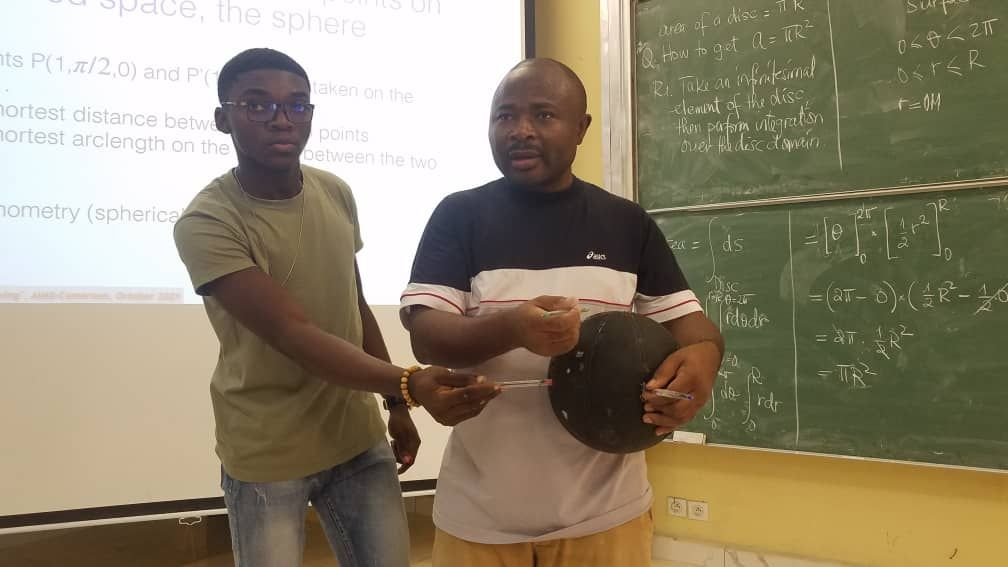November 12, 2021

# Dr. ETIENNE WAMBA Lectures Students on Concepts in Physics and Physical Problem Solving

Our 9th cohort students are this week completing the Skills Phase of the First Block in the academic calendar with a course titled “Concepts in Physics and  Physical Problem Solving” taught by Dr. Etienne Wamba.

He has lectured the students on The Classical Motion with Newton’s Second Law & Euler-Lagrange equation; The Electric Field with Maxwell’s equations; as well as Elements of Vector Calculus.

In the Vector Calculus lectures, Dr. Etienne Wamba introduced various types of The Coordinate Systems such as The Cartesian Coordinates, The Cylindrical Coordinates and The Spherical Polar Coordinates. The problem used to illustrate these was calculating the distance between two points on a curved space, the sphere. Other illustrations used by the lecturer, assisted by tutor Sylvain Wagoum, included calculating distance on flat space.

The students have also learned Vector Calculus in Kinematics and The Differential Field Operators.